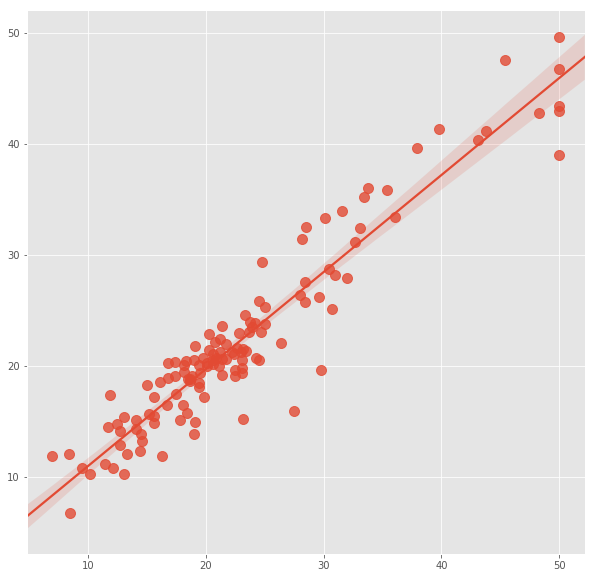How to use GradientBoosting Classifier and Regressor in Python?
0

# How to use GradientBoosting Classifier and Regressor in Python?

This recipe helps you use GradientBoosting Classifier and Regressor in Python
In :
```## How to use GradientBoosting Classifier and Regressor in Python
def Snippet_164():
print()
print(format('How to use GradientBoosting Classifier and Regressor in Python','*^82'))

import warnings
warnings.filterwarnings("ignore")

from sklearn import datasets
from sklearn import metrics
from sklearn.model_selection import train_test_split
import matplotlib.pyplot as plt
import seaborn as sns

plt.style.use('ggplot')

X = dataset.data; y = dataset.target
X_train, X_test, y_train, y_test = train_test_split(X, y, test_size=0.25)

# fit a CART model to the data
model.fit(X_train, y_train)
print(); print(model)

# make predictions
expected_y  = y_test
predicted_y = model.predict(X_test)

# summarize the fit of the model
print(); print(metrics.classification_report(expected_y, predicted_y))
print(); print(metrics.confusion_matrix(expected_y, predicted_y))

X = dataset.data; y = dataset.target
X_train, X_test, y_train, y_test = train_test_split(X, y, test_size=0.25)

# fit a CART model to the data
model.fit(X_train, y_train)
print(); print(model)

# make predictions
expected_y  = y_test
predicted_y = model.predict(X_test)

# summarize the fit of the model
print(); print(metrics.r2_score(expected_y, predicted_y))
print(); print(metrics.mean_squared_log_error(expected_y, predicted_y))

# plot regression
plt.figure(figsize=(10,10))
sns.regplot(expected_y, predicted_y, fit_reg=True, scatter_kws={"s": 100})

Snippet_164()
```
```**********How to use GradientBoosting Classifier and Regressor in Python**********

learning_rate=0.1, loss='deviance', max_depth=3,
max_features=None, max_leaf_nodes=None,
min_impurity_decrease=0.0, min_impurity_split=None,
min_samples_leaf=1, min_samples_split=2,
min_weight_fraction_leaf=0.0, n_estimators=100,
n_iter_no_change=None, presort='auto', random_state=None,
subsample=1.0, tol=0.0001, validation_fraction=0.1,
verbose=0, warm_start=False)

precision    recall  f1-score   support

0       1.00      1.00      1.00        55
1       1.00      1.00      1.00        88

micro avg       1.00      1.00      1.00       143
macro avg       1.00      1.00      1.00       143
weighted avg       1.00      1.00      1.00       143

[[55  0]
[ 0 88]]